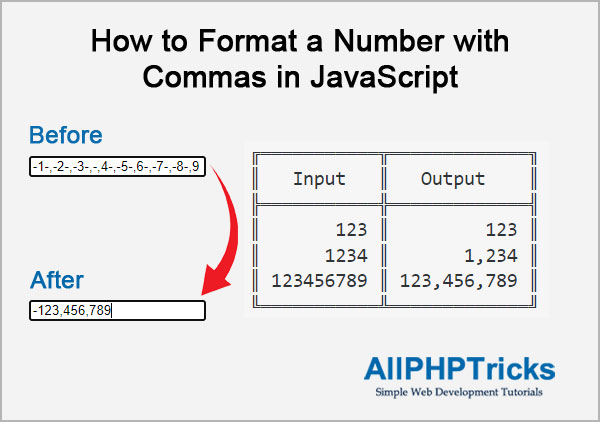# How to Format a Number with Commas in JavaScriptIn this tutorial, I will show you how to format a number with commas in JavaScript. When working with the application that is taking a large input of numbers, so it is better to format this large number into input field using JavaScript, so that user can easily understand the figure/amount in human readable format.

We can make it easily in human readable format by adding the commas in the large numbers, in this way anyone can understand what this large amount is.

We can use JavaScript to format number with commas and minus sign (-) if exist in the amount.

Although, there are several methods to achieve this, some of them are JavaScript built in functions and some are custom regular expressions.

I will focus on custom regular expressions as I believe in this method, we have more control to the code and we can do whatever we want in the code.

## Steps of How to Format a Number with Commas in JavaScript

To format a number with commas in JavaScript, we need to follow the below steps.

1. Write an HTML Markup
2. Write a JavaScript Code

### 1. Write an HTML Markup

Create an index.html file and paste the following HTML code in its body.

``````<label>Enter Amount 1:</label>

<input type="text" name="amount1" id="amount1"
class="numbers" placeholder="Amount 1"
onblur="numberWithCommas(this)">``````

As you can see above that I am using onblur HTML event attribute which executes a JavaScript function numberWithCommas(this) along with this in function parameter, when the input element loses focus.

I have additionally applied a numbers class which will use for validation purpose and accept only numbers, commas and minus sign (-) in the input field.

### 2. Write a JavaScript Code

Now, write the JavaScript function & include jQuery library for numbers class validation. You can add this JavaScript code in the footer section before closing of html body.

``````<script src="js/jquery.min.js"></script>
<script>

function numberWithCommas(field) {
// Get the input field ID
let element = document.getElementById(field.id);
// Replace all the commas of input field value with empty value
// And assign it to amount variable
let amount = element.value.replace(/,/g, '');

if(amount.charAt(0)=='-'){
// if amount contains (-) sign, remove first character then replace all
// (-) signs from the amount with empty value & concate (-) in amount
amount = '-'+amount.substring(1).replace(/-/g, '');
}else{
// Replace all (-) signs from the amount with empty value
amount = amount.replace(/-/g, '');
}
// First add commas into the amount then update the input field value
element.value =
amount.toString().replace(/\B(?<!\.\d*)(?=(\d{3})+(?!\d))/g, ",");
}

// Accept Only Numbers, Commas & (-) Minus Sign
\$(".numbers").on("keypress keyup blur",function (event) {
\$(this).val(\$(this).val().replace(/[^-\d,]+/g, ""));
if ((event.which < 48 || event.which > 57) &&
event.which!=44  && event.which!=45 ) {
event.preventDefault();
return false;
}
});
});
</script>``````

The above JavaScript code contains numberWithCommas function which formats a number with commas, but I want to validate that input field should accept only numbers, commas and (-) sign, therefore I created another script below the numberWithCommas function.

I prefer to validate input fields so that we get the the data in correct form.

### Conclusion

As you can see, it is very easy to format a number with commas in JavaScript, this is very useful when working with finance related applications. All you need is just a JavaScript function and you can call it to any number of input fields in the onblur event handler.

If you found this tutorial helpful, share it with your friends and developers group.

I spent several hours to create this tutorial, if you want to say thanks so like my page on Facebook, Twitter and share it.

Facebook Official Page: All PHP Tricks

Twitter Official Page: All PHP TricksArticle By
Javed Ur Rehman is a passionate blogger and web developer, he loves to share web development tutorials and blogging tips. He usually writes about HTML, CSS, JavaScript, Jquery, Ajax, PHP and MySQL.